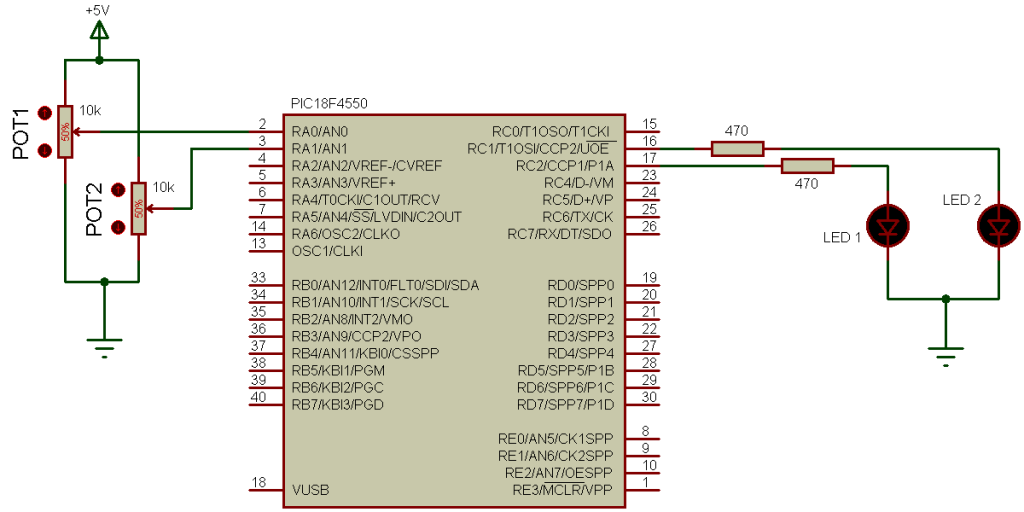# PIC18F4550 PWM example using CCS C compiler

PIC18F4550 has one CCP module and one ECCP (Enhanced CCP) module, the CCP module which CCP2 can generate a PWM signal on pin RC1, and ECCP which is CCP1 has an enhanced PWM capabilities, but this ECCP can work as a simple CCP. In this topic we are going to see how to use CCP1 (not enhanced CCP) and CCP2 to generate PWM signals on RC2 and RC1 respectively.

First we have to configure the CCP module to run as a PWM using the following CCS commands:
setup_ccp1(CCP_PWM);                // Configure CCP1 as a PWM
setup_ccp2(CCP_PWM);                // Configure CCP2 as a PWM
Then we have to use Timer2 to setup the pwm frequency.
Use the following equation to compute the pwm frequency:
PWM period = [(PR2) + 1] • 4 • Tosc • (TMR2 Prescale Value)
Where PWM frequency is defined as 1/[PWM period].
Tosc = 1/(MCU_frequency)
TMR2 Prescale Value can be 1, 4 or 16.

For example forPR2 = 250 , microcontroller frequency = 8MHz and Prescale = 16 we get a PWM frequency of 500Hz.
The CCS Timer2 configuration has the following form:
setup_timer_2(mode,  period, postscale);

where: mode is TMR2 Prescale Value, period is PR2 and postscaler is not used in the determination of the PWM frequency (keep it 1).
Previous example gives the following Timer2 configuration command:
setup_timer_2(T2_DIV_BY_16, 250, 1);
The last CCS command is the PWM duty cycle command:
set_pwm1_duty(value);